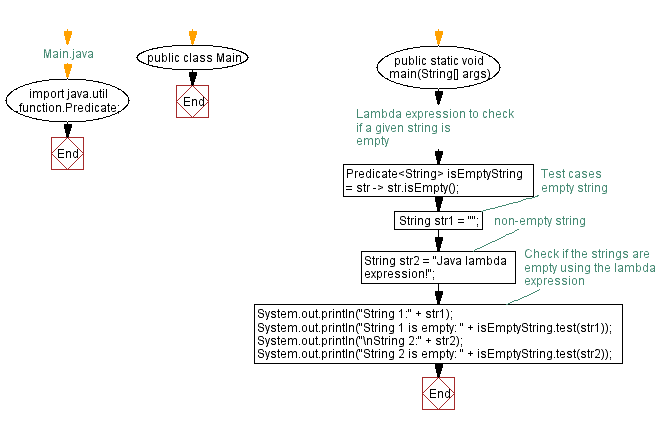# Java Lambda expression - Check if string is empty

## Java Lambda Program: Exercise-2 with Solution

Write a Java program to implement a lambda expression to check if a given string is empty.

Sample Solution:

Java Code:

``````// Main.java
import java.util.function.Predicate;

public class Main {
public static void main(String[] args) {
// Lambda expression to check if a given string is empty
Predicate isEmptyString = str -> str.isEmpty();

// Test cases
String str1 = ""; // empty string
String str2 = "Java lambda expression!"; // non-empty string

// Check if the strings are empty using the lambda expression
System.out.println("String 1:" + str1);
System.out.println("String 1 is empty: " + isEmptyString.test(str1));
System.out.println("\nString 2:" + str2);
System.out.println("String 2 is empty: " + isEmptyString.test(str2));
}
}
``````

Sample Output:

```String 1:
String 1 is empty: true

String 2:Java lambda expression!
String 2 is empty: false
```

Explanation:

In the above exercise, we define a Predicate functional interface with a lambda expression to check if a given string is empty. The lambda expression uses the isEmpty() method of the String class to determine if the string is empty.

To check if the strings are empty, we create two test cases, str1 and str2, and use the test() method of the Predicate interface. On the console, the results are displayed.

Flowchart:Live Demo:

Java Code Editor:

Improve this sample solution and post your code through Disqus

Java Lambda Exercises Previous: Sum of two integers.
Java Lambda Exercises Next: Convert list of strings to uppercase and lowercase using Lambda expression in Java.

What is the difficulty level of this exercise?

Test your Programming skills with w3resource's quiz.

﻿